Name:    Quiz 6.1-6.4

Multiple Choice
Identify the choice that best completes the statement or answers the question.

Write the decimal as a percent.

1.

2.95
 a. 0.0295% c. 295% b. 29.5% d. 2.95%

2.

0.58
 a. 580% c. 58% b. 5.8% d. 0.58%

3.

0.532
 a. 53.2% c. 5.32% b. 532% d. 0.532%

Write the percent as a decimal.

4.

40%
 a. 0.04 c. 4 b. 40 d. 0.4

5.

35.8%
 a. 35.8 c. 0.358 b. 3.58 d. 0.0358

6.

0.03%
 a. 0.03 c. 0.0003 b. 3 d. 0.003

Use a number line to order the numbers from least to greatest.

7.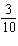, 0.34,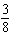, 27%
 a., 0.34, 27%,c. 27%, 0.34,,b. 27%,, 0.34,d., 0.34,, 27%

8.

74%,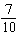, 0.68
 a. 0.68,, 74% c. 74%,, 0.68 b. 0.68, 74%,d., 74%, 0.68

Tell which number is greater.

9.

64%,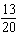a. 64% b.c. They are equal.

10.

30%, 0.03
 a. 0.03 b. They are equal. c. 30%

Write and solve a proportion to answer the question.

11.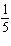is 40% of what number?
 a.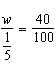; w =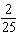c.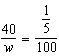; w =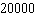b.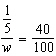; w =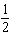d.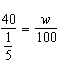; w =12.

What number is 25% of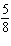?
 a.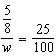; w =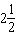c.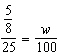; w =b.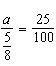; a =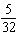d.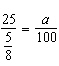; a =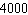13.

What percent of 50 is 22?
 a.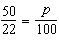; p = 227.3 c.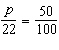; p = 11 b.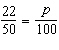; p = 44 d.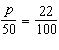; p = 11

Write and solve an equation to answer the question.

14.

48 is what percent of 80?
 a. 60% c. 64% b. 166.67% d. 59%

15.

4% of what number is 40?
 a. 950 c. 1000 b. 1.6 d. 1025

16.

A basketball player made 75 baskets in a season. Of these, 8% were three-point shots. How many three-point shots did the player make?
 a. 5 c. 6 b. 69 d. 7

Numeric Response

1.

You earn 4.2% on your savings account. Write this percent as a decimal.

2.

A store owner uses a selling price of 125% of the cost of an item. If a pair of jeans has a sales price of \$52.50, what cost does the store owner pay for the pair of jeans.